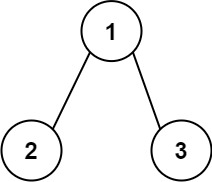129. Sum Root to Leaf Numbers
Medium
6.6K
100

You are given the `root` of a binary tree containing digits from `0` to `9` only.

Each root-to-leaf path in the tree represents a number.

• For example, the root-to-leaf path `1 -> 2 -> 3` represents the number `123`.

Return the total sum of all root-to-leaf numbers. Test cases are generated so that the answer will fit in a 32-bit integer.

A leaf node is a node with no children.

Example 1:```Input: root = [1,2,3]
Output: 25
Explanation:
The root-to-leaf path `1->2` represents the number `12`.
The root-to-leaf path `1->3` represents the number `13`.
Therefore, sum = 12 + 13 = `25`.
```

Example 2:```Input: root = [4,9,0,5,1]
Output: 1026
Explanation:
The root-to-leaf path `4->9->5` represents the number 495.
The root-to-leaf path `4->9->1` represents the number 491.
The root-to-leaf path `4->0` represents the number 40.
Therefore, sum = 495 + 491 + 40 = `1026`.
```

Constraints:

• The number of nodes in the tree is in the range `[1, 1000]`.
• `0 <= Node.val <= 9`
• The depth of the tree will not exceed `10`.
Accepted
602.5K
Submissions
987.4K
Acceptance Rate
61.0%

Seen this question in a real interview before?
1/4
Yes
No

Discussion (0)

Related Topics